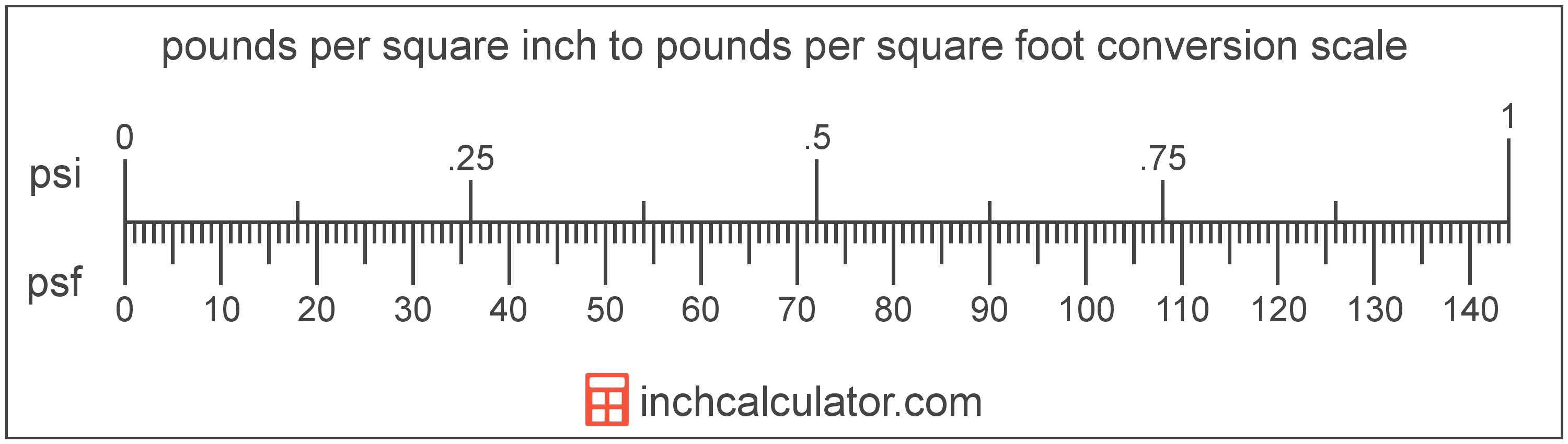# Pounds per Square Inch to Pounds per Square Foot Converter

Enter the pressure in pounds per square inch below to get the value converted to pounds per square foot.

Results in Pounds per Square Foot:1 psi = 144 psf

Do you want to convert pounds per square foot to pounds per square inch?

## How to Convert Pounds per Square Inch to Pounds per Square Foot

To convert a measurement in pounds per square inch to a measurement in pounds per square foot, multiply the pressure by the following conversion ratio: 144 pounds per square foot/pound per square inch.

Since one pound per square inch is equal to 144 pounds per square foot, you can use this simple formula to convert:

pounds per square foot = pounds per square inch × 144

The pressure in pounds per square foot is equal to the pressure in pounds per square inch multiplied by 144.

For example, here's how to convert 5 pounds per square inch to pounds per square foot using the formula above.
pounds per square foot = (5 psi × 144) = 720 psfPounds per square inch and pounds per square foot are both units used to measure pressure. Keep reading to learn more about each unit of measure.

## What Are Pounds per Square Inch?

One pound per square inch is the pressure of equal to one pound-force per square inch.

The pound per square inch is a US customary and imperial unit of pressure. A pound per square inch is sometimes also referred to as a pound-force per square inch. Pounds per square inch can be abbreviated as psi; for example, 1 pound per square inch can be written as 1 psi.

PSI can be expressed using the formula:
1 psi = 1 lbf / in2

Pressure in pounds per square inch are equal to the pound-force divided by the area in square inches.

## What Are Pounds per Square Foot?

One pound per square foot is the pressure of equal to one pound-force per square foot.

The pound per square foot is a US customary and imperial unit of pressure. Pounds per square foot can be abbreviated as psf; for example, 1 pound per square foot can be written as 1 psf.

PSF can be expressed using the formula:
1 psf = 1 lbf / ft2

Pressure in pounds per square foot are equal to the pound-force divided by the area in square feet.

## Pound per Square Inch to Pound per Square Foot Conversion Table

Table showing various pound per square inch measurements converted to pounds per square foot.
Pounds Per Square Inch Pounds Per Square Foot
1 psi 144 psf
2 psi 288 psf
3 psi 432 psf
4 psi 576 psf
5 psi 720 psf
6 psi 864 psf
7 psi 1,008 psf
8 psi 1,152 psf
9 psi 1,296 psf
10 psi 1,440 psf
11 psi 1,584 psf
12 psi 1,728 psf
13 psi 1,872 psf
14 psi 2,016 psf
15 psi 2,160 psf
16 psi 2,304 psf
17 psi 2,448 psf
18 psi 2,592 psf
19 psi 2,736 psf
20 psi 2,880 psf
21 psi 3,024 psf
22 psi 3,168 psf
23 psi 3,312 psf
24 psi 3,456 psf
25 psi 3,600 psf
26 psi 3,744 psf
27 psi 3,888 psf
28 psi 4,032 psf
29 psi 4,176 psf
30 psi 4,320 psf
31 psi 4,464 psf
32 psi 4,608 psf
33 psi 4,752 psf
34 psi 4,896 psf
35 psi 5,040 psf
36 psi 5,184 psf
37 psi 5,328 psf
38 psi 5,472 psf
39 psi 5,616 psf
40 psi 5,760 psf